# Pre-Algebra : One-Step Equations with Integers

## Example Questions

← Previous 1 3 4 5 6 7

### Example Question #1 : One Step Equations

Solve for: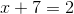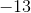Explanation:

Isolate the variable to one side.

Subtractfrom both sides: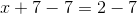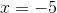### Example Question #1 : One Step Equations With Integers

Solve for: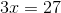Explanation:

We need to isolateby dividing by 3.

Remember, what you do on one side, you must do on the other side.

Since you dividedby, you also have to divdeby: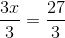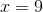### Example Question #1 : One Step Equations With Integers

Solve for: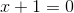Explanation:

To solve this you must isolate yourby subtracting 1 from both sides to get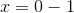or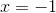### Example Question #1 : One Step Equations

Solve for x: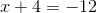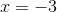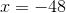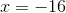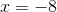Explanation:

Step 1: isolate x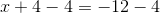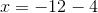Step 2: solveIf it's helpful, when you subtract a positive integer from a negative integer, you can think of it in terms of absolute value: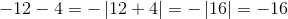### Example Question #5 : One Step Equations With Integers

Solve for: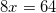4

8

9

6

7

8

Explanation:

To solve this equation, isolateby dividing both sides of the equation by 8: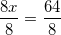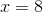### Example Question #6 : One Step Equations With Integers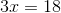Explanation:

To solve this equation, isolate the variable. Since 3x is a multiplication operation, do the opposite (division) to remove the 3. Keep in mind that you must do the same step on each side of the equation every time you change something.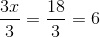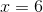### Example Question #1 : One Step Equations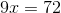Explanation:

To solve this equation, you must isolate the variable. In order to do this, do the opposite operation (division) to move the 9 to the other side of the equation. Keep in mind you must do the same step on each side of the equation every time you change something.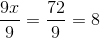Your result shoud look like this:### Example Question #8 : One Step Equations With Integers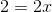Explanation:

Isolate the variable by dividng both sides by 2: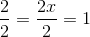### Example Question #1 : One Step Equations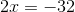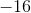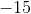Explanation:

To solve this equation, isolate the variable. To do this, move the 2 to the other side of the equation. Do this by doing the opposite operation (division). Keep in mind you must do the same step on each side of the equation every time you change something.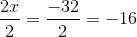### Example Question #1 : One Step Equations With Integers

Solve for: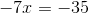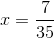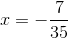Explanation: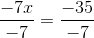← Previous 1 3 4 5 6 7

### All Pre-Algebra Resources